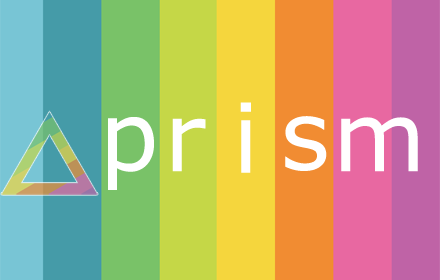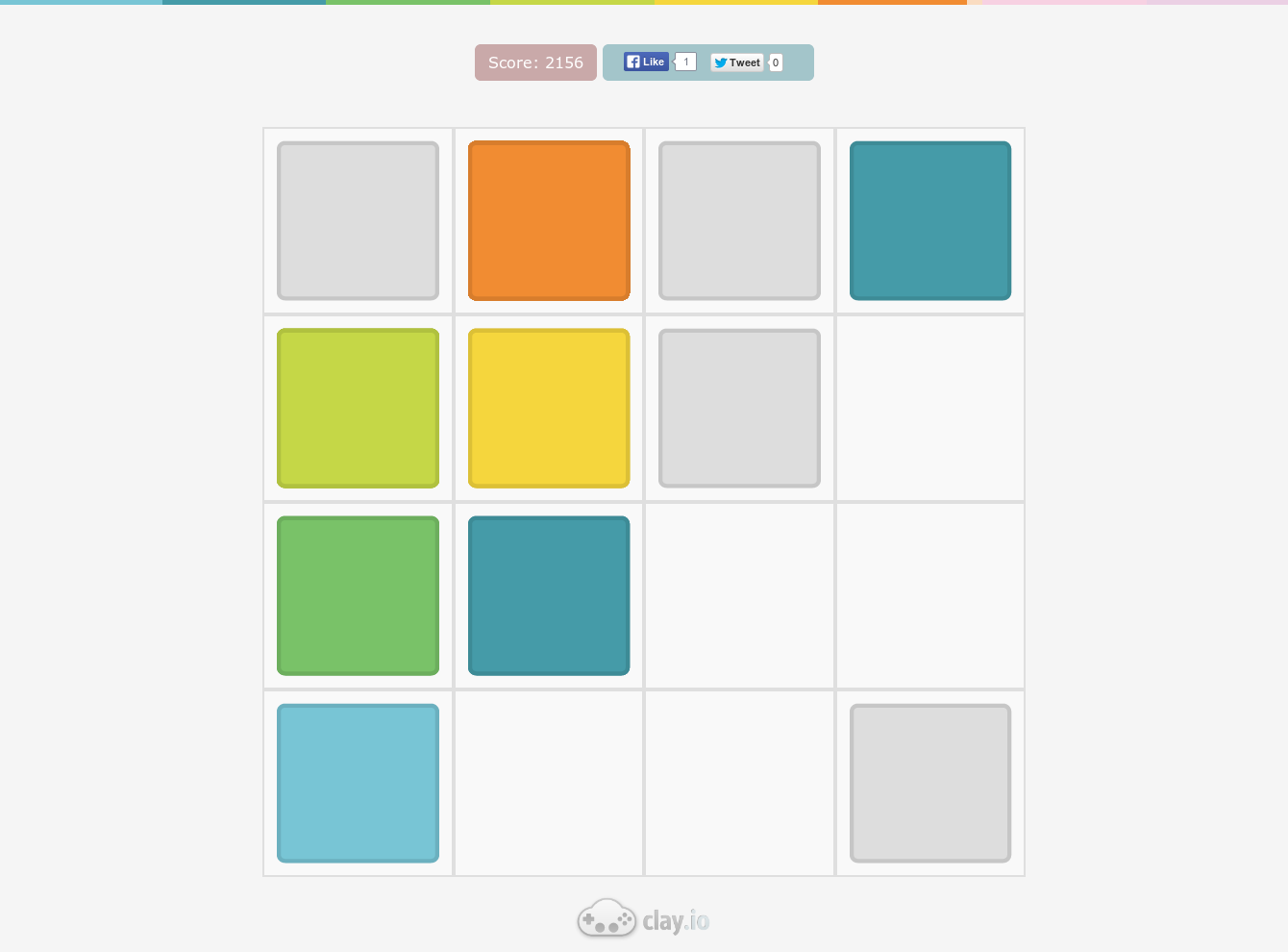# PrismPrism (source) is a 2048 clone, using colors instead of numbers. It was created by Austin and myself in about a day, mostly for the purposes of getting to know each other better (this was before I joined Clay.io) as well as to show investors that we could produce content quickly. We have since changed course, and are focusing on the marketplace instead of being a first-party app developer, but it was still a valuable experience.

My very first commit (with code) implemented the board logic of the game (this was a clone, not a fork of 2048), with tests! These tests were invaluable for helping us quickly iterate on the game mechanics, without spending time dealing with game logic errors.#### Game Logic

To be fair, I should have looked at how the game logic was implemented in the original 2048 game before writing it myself, but then again that wouldn't have been nearly as fun.

Here is the logic, commented for clarity:

``````this._move = function(dir) {

// Directions to move values
var keymap = {
up: [-1, 0],
down: [1, 0],
left: [0, -1],
right: [0, 1]
}

var grid = this.grid
var diff = keymap[dir]

// These represent the order in which the rows/columns will be traversed
var rows = _.range(0, 4)
var cols = _.range(0, 4)

// Change traversal order to make the movement logic simpler
if(dir === 'down') {
rows.reverse()
}
if(dir === 'right') {
cols.reverse()
}

// In each row, take each column and if it has a value in it, move it
// Above, we made sure to always travel along the path of cell movement
// That way we can avoid any recursion
// The logic is similar to a sideways waterfall

// ----                ----
// --a-   -> left ->   a---
// ----                ----
// ----                ----

for(var r=0;r<rows.length;r++) {
for(var c=0;c<cols.length;c++) {
var row = rows[r], col = cols[c]
if (!grid[row] || !grid[row][col]) return

// While the current cell has an item, and can move into the next cell
while (grid[row + diff] && (grid[row + diff][col + diff] === 0 ||
grid[row][col] === grid[row + diff][col + diff])) {

var combine = grid[row][col]
if (grid[row][col] === grid[row + diff][col + diff]) {

// Hack, if something has been combined, it has 0.1 added to it temporarily
combine = grid[row][col] + 1.1
}

// Update previous cell
grid[row][col] = 0
row += diff
col += diff

// Update new cell
grid[row][col] = combine
}
}
}

// This un-does the hack
this.grid = _.map(grid, function(row) {
return _.map(row, Math.floor.bind(Math))
})
}
``````

#### Gestures

In order to get Hammer.js to work on older devices I had to bind to the `dragX` events instead of the `swipeX` events:

``````Hammer(window, {
drag_min_distance:5,
drag_block_horizontal:true,
drag_block_vertical:true
}).on("dragleft", function(e) {
e.preventDefault()
e.gesture.preventDefault()
move='left'
}).on("dragright", function(e) {
e.preventDefault()
e.gesture.preventDefault()
move='right'
}).on("dragup", function(e) {
e.preventDefault()
e.gesture.preventDefault()
move='up'
}).on("dragdown", function(e) {
e.preventDefault()
e.gesture.preventDefault()
move='down'
}).on('dragend', function(e) {
GAME.board.move(move)
})
``````

#### Side Note

During the development, I got to use my micro-events library, which I wrote in contest with minivents.js a while back.

The entire thing is 345 bytes un-gzipped (with further optimizations still possible):

``````(function(){var d={},c=0,a,b;this.Events={on:function(a,c,b){d[a]=d[a]||[];d[a].push({f:c,c:b})},off:function(b,e){a=d[b]||[];if(!e)return a.length=0;for(c=a.length;0<=--c;)e==a[c].f&&a.splice(c,1)},emit:function(){b=Array.apply([],arguments);a=d[b.shift()]||[];b=binstanceof Array&&b||b;for(c=a.length;0<=--c;)a[c].f.apply(a[c].c,b)}}})()
``````

It turns out that you can convert `arguments` to an array by simply calling apply:

``````Array.apply([], arguments)
``````